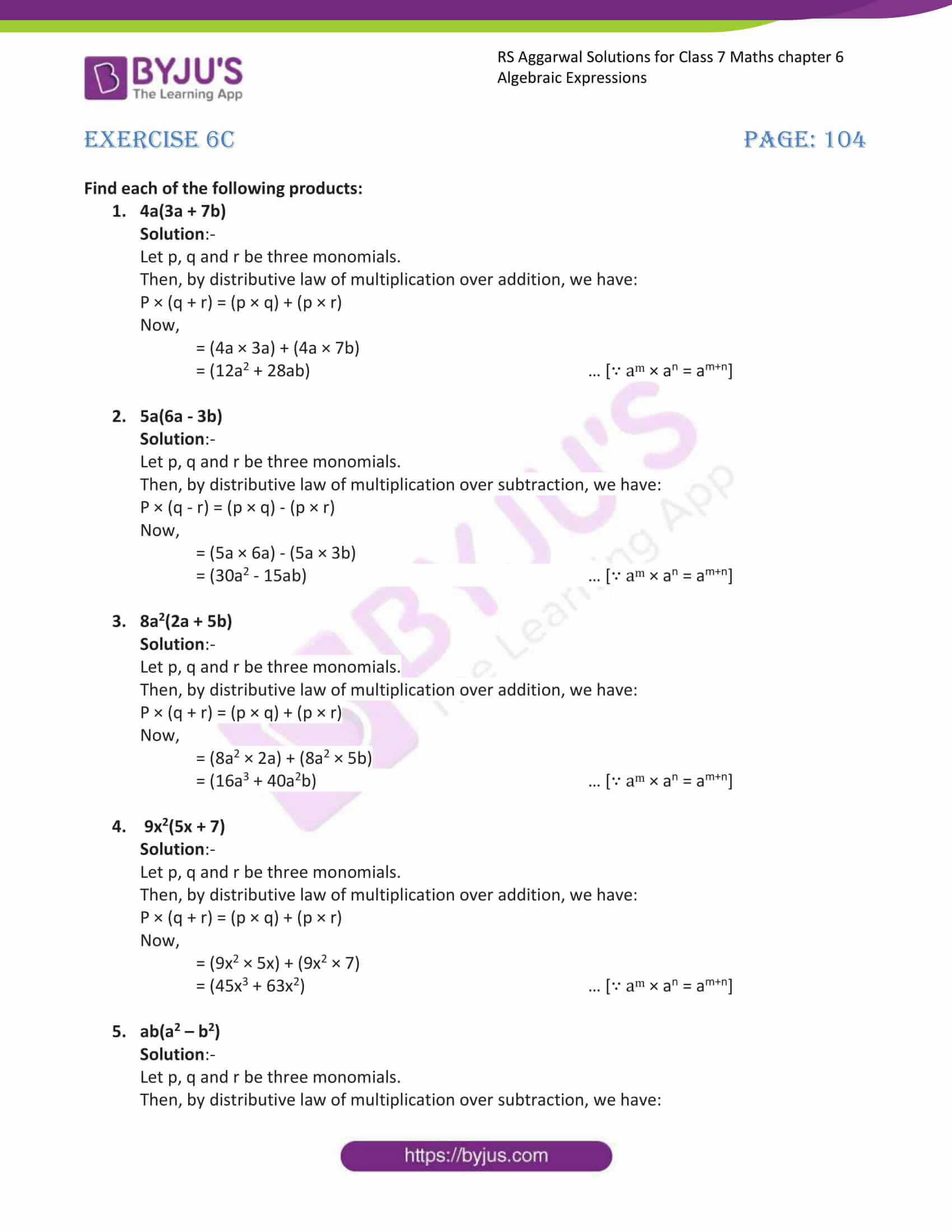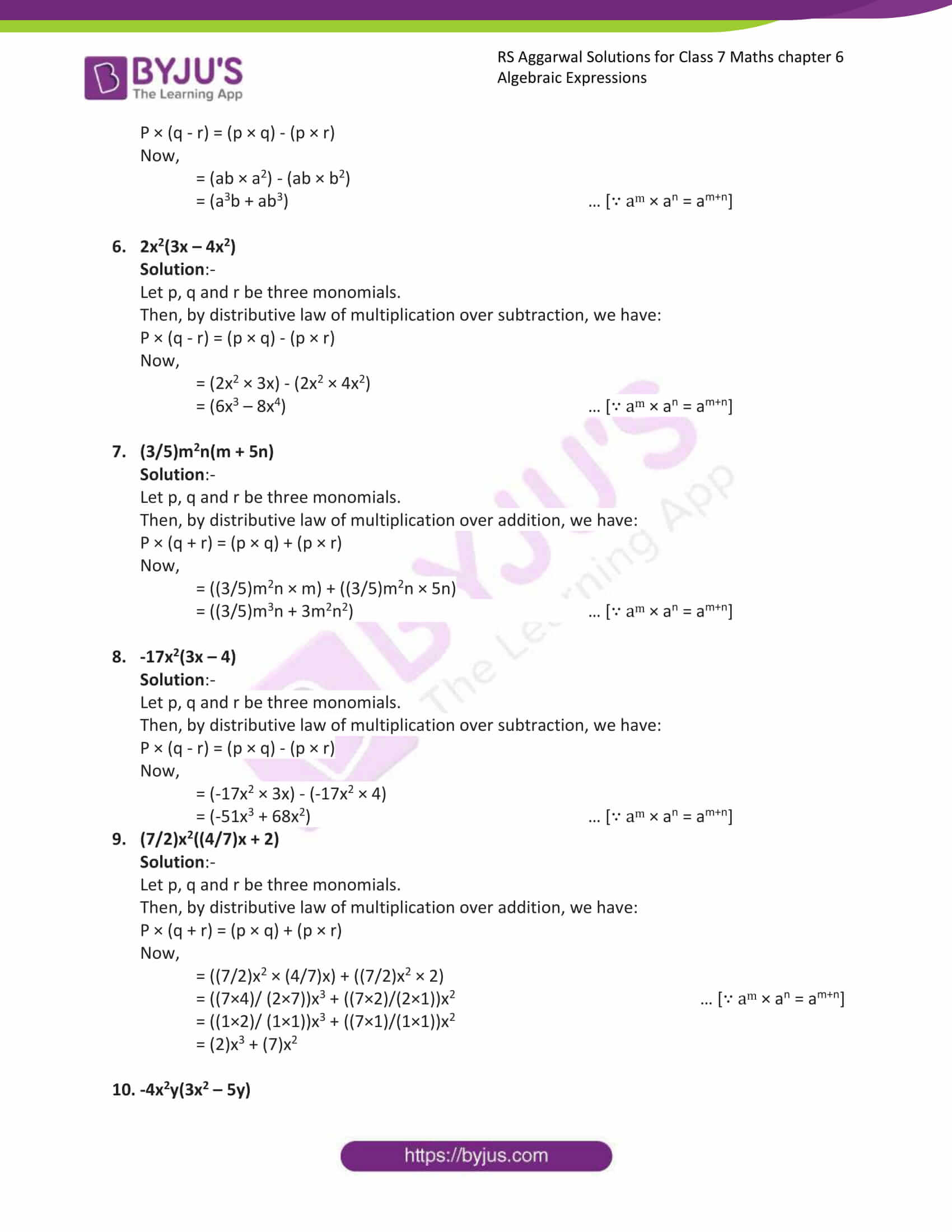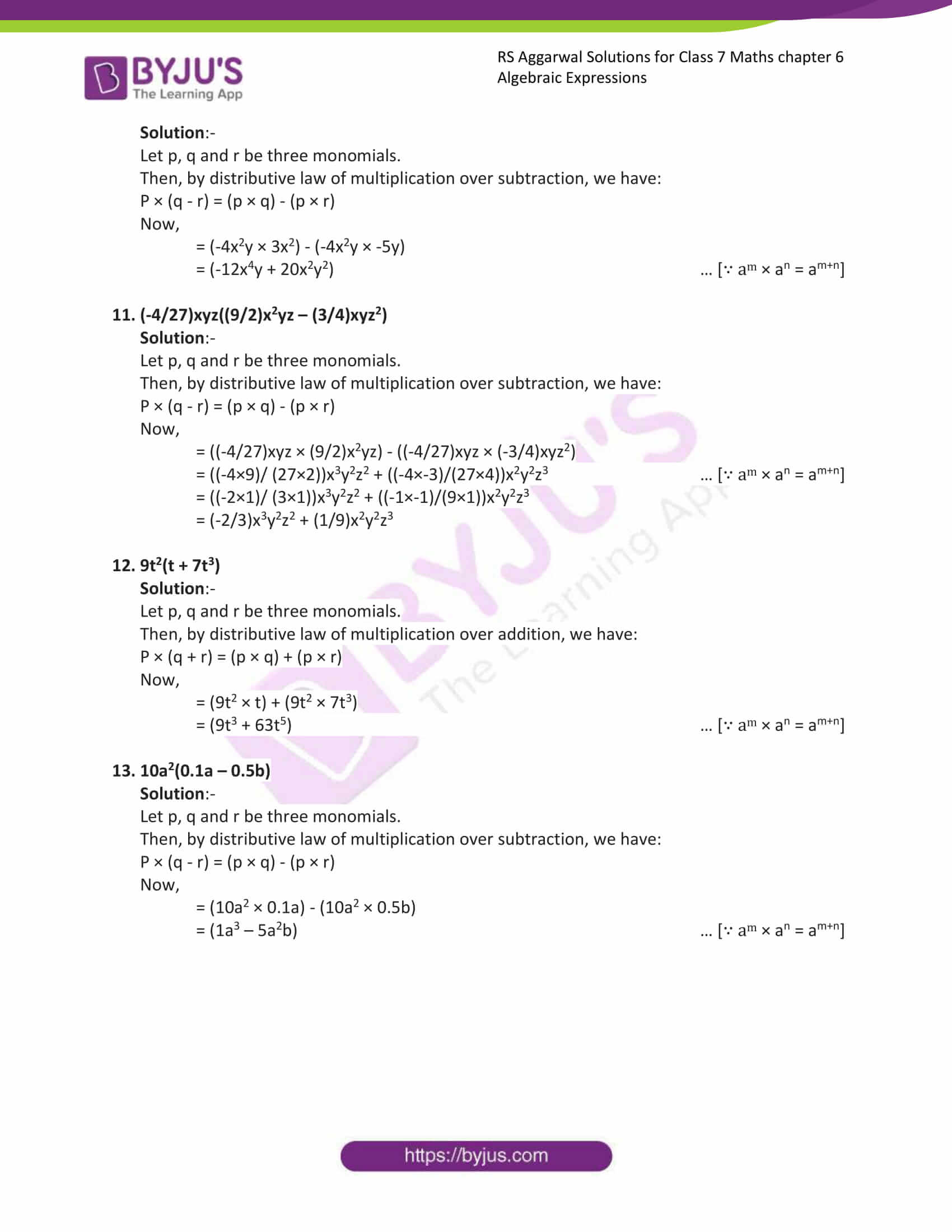# RS Aggarwal Solutions for Class 7 Maths Exercise 6C Chapter 6 Algebraic Expressions

RS Aggarwal Solutions for Class 7 Maths Exercise 6C Chapter 6 Algebraic Expressions in simple PDF are given here. Multiplication of a monomial and a binomial is the topic covered in this exercise of RS Aggarwal Solutions for Class 7 Chapter 6. The main aim is to help students understand and crack these problems. We at BYJU’S have prepared the RS Aggarwal Solutions for Class 7 Maths Chapter 6 Algebraic Expressions. By practising these solutions, students can get good marks in Maths.

## Download the PDF of RS Aggarwal Solutions For Class 7 Maths Chapter 6 Algebraic Expressions – Exercise 6C### Access answers to Maths RS Aggarwal Solutions for Class 7 Chapter 6 – Algebraic Expressions Exercise 6C

Find each of the following products:

1. 4a(3a + 7b)

Solution:-

Let p, q and r be three monomials.

Then, by distributive law of multiplication over addition, we have:

P × (q + r) = (p × q) + (p × r)

Now,

= (4a × 3a) + (4a × 7b)

= (12a2 + 28ab) … [∵ am × an = am+n]

2. 5a(6a – 3b)

Solution:-

Let p, q and r be three monomials.

Then, by distributive law of multiplication over subtraction, we have:

P × (q – r) = (p × q) – (p × r)

Now,

= (5a × 6a) – (5a × 3b)

= (30a2 – 15ab) … [∵ am × an = am+n]

3. 8a2(2a + 5b)

Solution:-

Let p, q and r be three monomials.

Then, by distributive law of multiplication over addition, we have:

P × (q + r) = (p × q) + (p × r)

Now,

= (8a2 × 2a) + (8a2 × 5b)

= (16a3 + 40a2b) … [∵ am × an = am+n]

4. 9x2(5x + 7)

Solution:-

Let p, q and r be three monomials.

Then, by distributive law of multiplication over addition, we have:

P × (q + r) = (p × q) + (p × r)

Now,

= (9x2 × 5x) + (9x2 × 7)

= (45x3 + 63x2) … [∵ am × an = am+n]

5. ab(a2 – b2)

Solution:-

Let p, q and r be three monomials.

Then, by distributive law of multiplication over subtraction, we have:

P × (q – r) = (p × q) – (p × r)

Now,

= (ab × a2) – (ab × b2)

= (a3b + ab3) … [∵ am × an = am+n]

6. 2x2(3x – 4x2)

Solution:-

Let p, q and r be three monomials.

Then, by distributive law of multiplication over subtraction, we have:

P × (q – r) = (p × q) – (p × r)

Now,

= (2x2 × 3x) – (2x2 × 4x2)

= (6x3 – 8x4) … [∵ am × an = am+n]

7.(3/5)m2n(m + 5n)

Solution:-

Let p, q and r be three monomials.

Then, by distributive law of multiplication over addition, we have:

P × (q + r) = (p × q) + (p × r)

Now,

= ((3/5)m2n × m) + ((3/5)m2n × 5n)

= ((3/5)m3n + 3m2n2) … [∵ am × an = am+n]

8. -17x2(3x – 4)

Solution:-

Let p, q and r be three monomials.

Then, by distributive law of multiplication over subtraction, we have:

P × (q – r) = (p × q) – (p × r)

Now,

= (-17x2 × 3x) – (-17x2 × 4)

= (-51x3 + 68x2) … [∵ am × an = am+n]

9. (7/2)x2((4/7)x + 2)

Solution:-

Let p, q and r be three monomials.

Then, by distributive law of multiplication over addition, we have:

P × (q + r) = (p × q) + (p × r)

Now,

= ((7/2)x2 × (4/7)x) + ((7/2)x2 × 2)

= ((7×4)/ (2×7))x3 + ((7×2)/(2×1))x2 … [∵ am × an = am+n]

= ((1×2)/ (1×1))x3 + ((7×1)/(1×1))x2

= (2)x3 + (7)x2

10. -4x2y(3x2 – 5y)

Solution:-

Let p, q and r be three monomials.

Then, by distributive law of multiplication over subtraction, we have:

P × (q – r) = (p × q) – (p × r)

Now,

= (-4x2y × 3x2) – (-4x2y × -5y)

= (-12x4y + 20x2y2) … [∵ am × an = am+n]

11. (-4/27)xyz((9/2)x2yz – (3/4)xyz2)

Solution:-

Let p, q and r be three monomials.

Then, by distributive law of multiplication over subtraction, we have:

P × (q – r) = (p × q) – (p × r)

Now,

= ((-4/27)xyz × (9/2)x2yz) – ((-4/27)xyz × (-3/4)xyz2)

= ((-4×9)/ (27×2))x3y2z2 + ((-4×-3)/(27×4))x2y2z3 … [∵ am × an = am+n]

= ((-2×1)/ (3×1))x3y2z2 + ((-1×-1)/(9×1))x2y2z3

= (-2/3)x3y2z2 + (1/9)x2y2z3

12. 9t2(t + 7t3)

Solution:-

Let p, q and r be three monomials.

Then, by distributive law of multiplication over addition, we have:

P × (q + r) = (p × q) + (p × r)

Now,

= (9t2 × t) + (9t2 × 7t3)

= (9t3 + 63t5) … [∵ am × an = am+n]

13. 10a2(0.1a – 0.5b)

Solution:-

Let p, q and r be three monomials.

Then, by distributive law of multiplication over subtraction, we have:

P × (q – r) = (p × q) – (p × r)

Now,

= (10a2 × 0.1a) – (10a2 × 0.5b)

= (1a3 – 5a2b) … [∵ am × an = am+n]

### Access other exercises of RS Aggarwal Solutions For Class 7 Chapter 6 – Algebraic Expressions

Exercise 6D Solutions